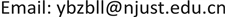1. 引言

2. 光栅延迟线的基本原理及其优点

T = 2 n e f f c ( z + L / 2 ) (2)

τ = 2 n e f f C c Δ λ (3)

3. 光控相控阵波束形成原理

φ = 2 π f ⋅ τ = 2 π f ⋅ n ⋅ l c (4)

Δ L θ B = d sin θ B (5)

Δ l = d sin θ B n (6)

τ = ( M − 1 ) ⋅ d sin θ B c (常量) (7)

Δ τ = d sin θ B c (8)

F ( θ ) = ∑ i = 0 N − 1 a i exp ( j i ( 2 π λ d sin θ − Δ ϕ B ) ) = ∑ i = 0 N − 1 a i exp ( j i ( 2 π λ d sin θ − 2 π λ d sin θ B ) ) (9)

θ B = arcsin ( λ 2 π d ⋅ Δ ϕ B ) (10)

F ( θ ) = ∑ i = 0 N − 1 a i exp ( j i ( 2 π λ d sin θ − Δ τ ) ) = ∑ i = 0 N − 1 a i exp ( j i ( 2 π λ d sin θ − d sin θ B c ) ) (11)

θ B = arcsin ( Δ τ ⋅ c d ) (12)

4. 光栅延迟线波束形成网络设计仿真

4.1. 光栅延迟线波束形成网络结构设计

4.2. 试验结果分析

5. 总结